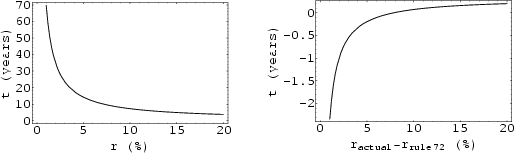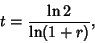## Rule of 72The time required for a given Principal to double (assumingConversion Period) for Compound Interest is given by solving(1)

or(2)

where Ln is the Natural Logarithm. This function can be approximated by the so-called rule of 72'':(3)

The above plots show the actual doubling time(left plot) and difference between actual and time calculated using the rule of 72 (right plot) as a function of the interest rate.